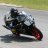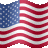# RevEngRSI channel on upper chart

S

#### skynetgen

##### Well-known member
Added smoothing and I like how it works on upper chart as channel with midline.

Code:
``````#skynetgen
#built from reverseneginner rsi. just added mid, upper and lower
#and removed "wilders" for simplicity. Remember widlers = 2xEMA (backtested this)

input length = 28; #14x = 28 ema
input price = close;
input rsiHi = 70.0;
input rsiMid = 50.0;
input rsiLow = 30.0;
input smooth=5;

script RevEngRSI
{
input length = 14;
input price = close;
input RSIValue=50.0;

def coeff = rsiValue / (100 - rsiValue);
def chg = price - price;
def  diff =  (length - 1) * (ExpAverage(Max(-chg, 0), length) * coeff - ExpAverage(Max(chg, 0), length)) / 2;
def value = price + if diff >= 0 then diff else diff / coeff;
plot RevEngRSI = compoundValue(1, value, Double.NaN);
}

plot pRSIHi= expaverage(revEngRSI(length,price, RSIHi),smooth);
plot pRSIMid=  expaverage(revEngRSI(length,price, RSIMid),smooth);
plot pRSILow=  expaverage(revEngRSI(length,price, RSILow),smooth);
pRsiHi.SetDefaultColor(GetColor(8));
pRsiMid.SetDefaultColor(GetColor(8));
pRsiLow.SetDefaultColor(GetColor(8));``````
https://tos.mx/9wOtgjQ

Last edited by a moderator:#### horserider

##### Well-known member
VIP
I had looked at the ReverseEngineered RSI in ToS. Decided not to use it. This looks like an improvement. Nice creative thinking,Enhanced RevEngRSI indicator (extension and alerts) Custom 8Major And Minor Wave Lines / Price Channel Labels for ThinkorSwim Indicators 14